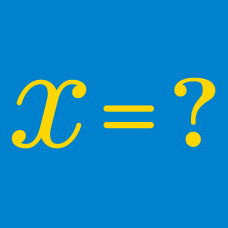Algebra

# Roots WarmupRaising a number to the $\frac{1}{2}$ power is the same as its square root.

Which of the following is equal to the expression above?

What is the value of

$\large \sqrt{ ( \color{#D61F06}-8\color{#333333}) ^ 2 } \hspace{.15cm} ?$

$\large \sqrt{\color{#69047E}{99} \times \color{#69047E}{99}+\color{#20A900}{199}} = \ \color{#D61F06} ?$

Note: There is a clever way to solve this problem without computing $99^2$.

$\Large \sqrt{ {\color{#D61F06} { 7}} \times \color{#3D99F6} {x} } = \color{#20A900} {49 }$

Solve for $x$.

$\sqrt{ \frac{ 1 } { x } } = 8, \ \ \ \ \ x = \ ?$

×